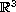Electron. J. Diff. Eqns., Vol. 2007(2007), No. 65, pp. 1-37.

### Local solvability of degenerate Monge-Ampère equations and applications to geometry Marcus A. Khuri

Abstract:
We consider two natural problems arising in geometry which are equivalent to the local solvability of specific equations of Monge-Ampère type. These are: the problem of locally prescribed Gaussian curvature for surfaces in, and the local isometric embedding problem for two-dimensional Riemannian manifolds. We prove a general local existence result for a large class of degenerate Monge-Ampère equations in the plane, and obtain as corollaries the existence of regular solutions to both problems, in the case that the Gaussian curvature vanishes and possesses a nonvanishing Hessian matrix at a critical point.

Submitted February 28, 2007. Published May 9, 2007.
Math Subject Classifications: 53B20, 53A05, 35M10.
Key Words: Local solvability; Monge-Ampère equations; isometric embeddings.

Show me the PDF file (434K), TEX file, and other files for this article.

 Marcus A. Khuri Department of Mathematics, Stony Brook University Stony Brook, NY 11794, USA email: khuri@math.sunysb.edu